ISEE Middle Level Math : How to find percentage

Example Questions

Example Question #101 : Percentage

Find the decimal equivalent of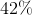.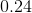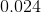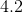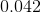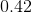Explanation:

To solve, divide: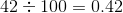Answer:Example Question #102 : Percentage

Find the decimal equivalent of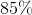.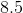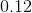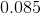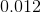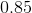Explanation:

To solve, divide: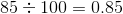Answer:Example Question #103 : Percentage

Find the decimal equivalent of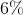.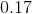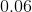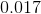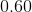None of theseExplanation:

To solve, divide: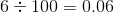Answer:Example Question #104 : Percentage

Find the decimal equivalent of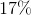.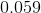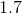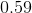Explanation:

To solve, divide: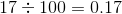Answer:Example Question #105 : Percentage

What is the equivalent of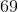%?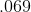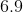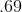Explanation:

To solve, divide: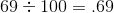Answer:Example Question #106 : Percentage

What is the equivalent of%?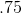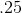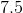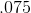Explanation:

To solve, divide: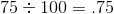Answer:Example Question #107 : Percentage

What is the equivalent of%?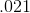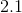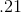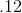Explanation:

To solve, divide: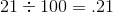Answer:Example Question #108 : Percentage

What is the equivalent of%?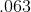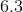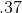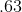Explanation:

To solve, divide: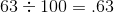Answer:Example Question #109 : Percentage

Find the decimal equivalent of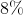.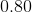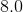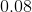None of these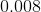Explanation:

To solve, divide: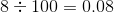Answer:Example Question #110 : Percentage

Find the decimal equivalent of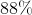.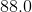None of these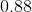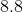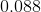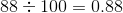Answer: